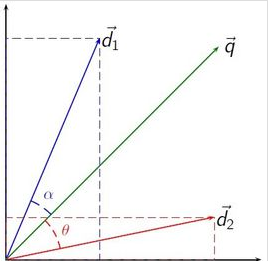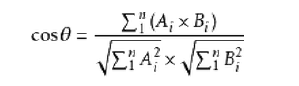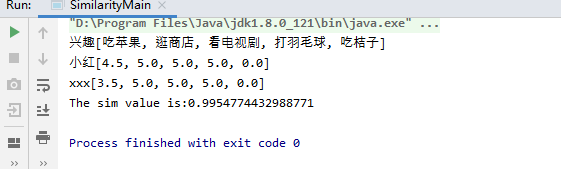• Matlab余弦相似度算法判断图片相似度并识别源代码 Matlab 余弦相似度 图像匹配 可直接运行 Matlab余弦相似度算法判断图片相似度并识别源代码 Matlab 余弦相似度 图像匹配 可直接运行
• Java 实现推荐系统 两个字符串 余弦相似度 算法
• 主要介绍了PHP数据分析引擎计算余弦相似度算法,结合具体实例形式分析了php计算余弦相似度的操作步骤与相关实现技巧,需要的朋友可以参考下
• （1） 基于用户的协同过滤算法 基于用户行为数据设计的推荐算法一般称为协同过滤算法。学术界对协同过滤算法 进行了深入研究，提出了很多方法，比如基于邻域的方法（neighborhood-based）、隐语义模型（latent ...
（1） 基于用户的协同过滤算法
基于用户行为数据设计的推荐算法一般称为协同过滤算法。学术界对协同过滤算法 进行了深入研究，提出了很多方法，比如基于邻域的方法（neighborhood-based）、隐语义模型（latent factor model）、基于图的随机游走算法（random walk on graph）等。 基于邻域的算法是推荐系统中最基本的算法，该算法不仅在学术界得到了深入研究，而且在 业界得到了广泛应用。基于邻域的算法分为两大类，一类是基于用户的协同过滤算法，另一类是 基于物品的协同过滤算法。 基于用户的协同过滤算法是推荐系统中最古老的算法，这个算法是1992年提出的，是用在了邮件过滤系统中，后面被用到了新闻过滤系统中。 简单的来说，在一个在线个性化推荐系统中，基于用户的协同过滤就是，当一个用户A需要个性化推荐 时，可以先找到和他有相似兴趣的其他用户，然后把那些用户喜欢的、而用户A没有听说过的物品推荐给A。这种方法称为基于用户的协同过滤算法。 协同过滤算法主要利用行为的相似度 计算兴趣的相似度。余弦相似度是一种计算方法。
（2） 余弦相似度原理 用向量空间中的两个向量夹角的余弦值作为衡量两个个体间差异大小的度量，值越接近1，就说明夹角角度越接近0°，也就是两个向量越相似，就叫做余弦相似，衡量的是两个变量在各个方向(属性)上的比例的相似度。
（3） 余弦相似度公式 给定用户u和用户v，令N(u)表示用户u曾经有过正反馈的物品集合，令N(v) 为用户v曾经有过正反馈的物品集合。 《推荐系统实践》书中的计算公式：（4） 推导公式的过程 二维变量余弦相似度的计算： 如图夹角为θ，图中c是一条辅助线：上述a、b、c边的长度分别为：根据余弦定理有:n维变量余弦相似度的计算：展开全文自动驾驶
• 这篇文章主要介绍了PHP数据分析引擎计算余弦相似度算法,结合具体实例形式分析了php计算余弦相似度的操作步骤与相关实现技巧,需要的朋友可以参考下本文实例讲述了PHP数据分析引擎计算余弦相似度算法。分享给大家供...

这篇文章主要介绍了PHP数据分析引擎计算余弦相似度算法,结合具体实例形式分析了php计算余弦相似度的操作步骤与相关实现技巧,需要的朋友可以参考下
本文实例讲述了PHP数据分析引擎计算余弦相似度算法。分享给大家供大家参考，具体如下：
关于余弦相似度的相关介绍可参考百度百科：余弦相似度
<?php /** * 数据分析引擎 * 分析向量的元素 必须和基准向量的元素一致，取最大个数，分析向量不足元素以0填补。 * 求出分析向量与基准向量的余弦值 * @author yu.guo@okhqb.com *//** * 获得向量的模 * @param unknown_type $array 传入分析数据的基准点的N维向量。|eg:array(1,1,1,1,1); */function getMarkMod($arrParam){ $strModDouble = 0; foreach($arrParam as $val){$strModDouble += $val *$val; } $strMod = sqrt($strModDouble); //是否需要保留小数点后几位 return $strMod;}/** * 获取标杆的元素个数 * @param unknown_type$arrParam * @return number */function getMarkLenth($arrParam){$intLenth = count($arrParam); return$intLenth;}/** * 对传入数组进行索引分配，基准点的索引必须为k，求夹角的向量索引必须为 'j'. * @param unknown_type $arrParam * @param unknown_type$index * @ruturn $arrBack */function handIndex($arrParam, $index = 'k'){ foreach($arrParam as $key =>$val){ $in =$index.$key;$arrBack[$in] =$val; } return $arrBack;}/** * * @param unknown_type$arrMark标杆向量数组(索引被处理过) * @param unknown_type $arrAnaly 分析向量数组 (索引被处理过) |array('j0'=>1,'j1'=>2....) * @param unknown_type$strMarkMod标杆向量的模 * @param unknown_type $intLenth 向量的长度 */function getCosine($arrMark, $arrAnaly,$strMarkMod ,$intLenth){$strVector = 0; $strCosine = 0; for($i = 0; $i <$intLenth; $i++){$strMarkVal = $arrMark['k'.$i]; $strAnalyVal =$arrAnaly['j'.$i];$strVector += $strMarkVal *$strAnalyVal; } $arrAnalyMod = getMarkMod($arrAnaly); //求分析向量的模 $strFenzi =$strVector; $strFenMu =$arrAnalyMod * $strMarkMod;$strCosine = $strFenzi /$strFenMu; if(0 !== (int)$strFenMu){$strCosine = $strFenzi /$strFenMu; } return \$strCosine;}?>

展开全文• 余弦相似度算法: 余弦相似性通过测量两个向量的夹角的余弦值来度量它们之间的相似性。0度角的余弦值是1，而其他任何角度的余弦值都不大于1；并且其最小值是-1。从而两个向量之间的角度的余弦值确定两个向量是否...
余弦相似度算法:
余弦相似性通过测量两个向量的夹角的余弦值来度量它们之间的相似性。0度角的余弦值是1，而其他任何角度的余弦值都不大于1；并且其最小值是-1。从而两个向量之间的角度的余弦值确定两个向量是否大致指向相同的方向。两个向量有相同的指向时，余弦相似度的值为1；两个向量夹角为90°时，余弦相似度的值为0；两个向量指向完全相反的方向时，余弦相似度的值为-1。这结果是与向量的长度无关的，仅仅与向量的指向方向相关。余弦相似度通常用于正空间，因此给出的值为-1到1之间。
坐标系表示：具体公式:具体实现(Java)：
import java.util.ArrayList;

public class SimilarityUtil {

public static double similarity(ArrayList va, ArrayList vb) {
if (va.size() > vb.size()) {
int temp = va.size() - vb.size();
for (int i = 0; i < temp; i++) {
}
} else if (va.size() < vb.size()) {
int temp = vb.size() - va.size();
for (int i = 0; i < temp; i++) {
}
}

int size = va.size();
double simVal = 0;

double num = 0;
double den = 1;
double powa_sum = 0;
double powb_sum = 0;
for (int i = 0; i < size; i++) {
double a = Double.parseDouble(va.get(i).toString());
double b = Double.parseDouble(vb.get(i).toString());

num = num + a * b;
powa_sum = powa_sum + (double) Math.pow(a, 2);
powb_sum = powb_sum + (double) Math.pow(b, 2);
}
double sqrta = (double) Math.sqrt(powa_sum);
double sqrtb = (double) Math.sqrt(powb_sum);
den = sqrta * sqrtb;

simVal = num / den;

return simVal;
}
}

案例分析:
姓名/兴趣  吃苹果  逛商店  看电视剧  打羽毛球  吃桔子  小红  3.5  5  5  5  0                    xxx  3.5  5  5  0  0
分别以小红和xxx的兴趣评分组成向量va和vb，运用多维余弦相似公式，设向量 A =
(A1,A2,...,An)，B = (B1,B2,...,Bn) ：计算得相似度为:0.9954774432988771
具体实现代码：
import java.util.ArrayList;

public class SimilarityMain {

public static double similarity(ArrayList va, ArrayList vb) {
if (va.size() > vb.size()) {
int temp = va.size() - vb.size();
for (int i = 0; i < temp; i++) {
}
} else if (va.size() < vb.size()) {
int temp = vb.size() - va.size();
for (int i = 0; i < temp; i++) {
}
}

int size = va.size();
double simVal = 0;

double num = 0;
double den = 1;
double powa_sum = 0;
double powb_sum = 0;
for (int i = 0; i < size; i++) {
double a = Double.parseDouble(va.get(i).toString());
double b = Double.parseDouble(vb.get(i).toString());

num = num + a * b;
powa_sum = powa_sum + (double) Math.pow(a, 2);
powb_sum = powb_sum + (double) Math.pow(b, 2);
}
double sqrta = (double) Math.sqrt(powa_sum);
double sqrtb = (double) Math.sqrt(powb_sum);
den = sqrta * sqrtb;

simVal = num / den;

return simVal;
}

public static void main(String[] args) {
String item[] = {"吃苹果", "逛商店", "看电视剧", "打羽毛球", "吃桔子"};
float a[] = {(float) 4.5, 5, 5, 5,0};
float b[] = {(float) 3.5, 5, 5, 5,0};
ArrayList vitem = new ArrayList();
ArrayList<Float> va = new ArrayList();
ArrayList<Float> vb = new ArrayList();
for (int i = 0; i < a.length; i++)
{
}
System.out.print("兴趣");
System.out.println(vitem);
System.out.print("小红");
System.out.println(va);
System.out.print("xxx");
System.out.println(vb);

SimilarityMain sim = new SimilarityMain();

double simVal = sim.similarity(va, vb);

System.out.println("The sim value is:" + simVal);
}

}

源码gitee地址:
https://gitee.com/jockhome/
展开全文• 基于内容推荐算法和余弦相似度算法的领导决策辅助信息系统.pdf
• 基于TF-IDF算法、余弦相似度算法实现相似文本推荐——文本相似度算法，主要应用于文本聚类、相似文本推荐等场景。设计说明使用jieba切词，设置自定义字典使用TF-IDF算法，找出文章的关键词；每篇文章各取出若干个...基于TF-IDF算法、余弦相似度算法实现相似文本推荐——文本相似度算法，主要应用于文本聚类、相似文本推荐等场景。
设计说明
使用jieba切词，设置自定义字典
使用TF-IDF算法，找出文章的关键词；
每篇文章各取出若干个关键词（比如20个），合并成一个集合，计算每篇文章对于这个集合中的词的词频（待优化:为了避免文章长度的差异，可以使用相对词频,）；
生成两篇文章各自的词频向量；
计算两个向量的余弦相似度，值越大就表示越相似。
实现说明
1）初始化
需要对原始文档做些简单的处理，在预处理结果文件中每一行记录一个文档，文档ID与文档内容通过一定分隔符分割（比如以:::间隔），写入txt文档，当然并非一定如此处理，此处可以根据实际使用，调整代码实现。
我选取了五则新闻，其中1,2,3为房地产板块新闻，4,5为NBA板块新闻。原始文本预处理
其余初始化内容详见代码注释。
class DocumentSimilarity:
def __init__(self):
#停词字典路径
self.stopword = 'Cstopword.dic'
#原始文档路径，每一行一个文档，文档ID与文档内容以:::间隔
self.DocumentFilePath = 'Document.txt'
#切词结果存放路径
self.CutWordFilePath = 'CutWordNews.txt'
#每个文档选取关键词个数
self.KeyWordsNum = 10
#推荐相似文档个数
self.DocumentSimilarityNum = 6
#切词结果，格式为{文档ID:切词结果，……}
self.CutWordDict = {}
#文档关键词字典，格式为{文档ID:{关键词：TF-IDF值,……},……}
self.DocumentKeywords = {}
#文档词频字典，格式为{文档ID:{切词：词频,……},……}
self.WordsFrequency = {}
# 导入自定义字典
2）使用jieba切词
之前在
Python 中文分词——jieba
文章中已详细介绍过，这里不做赘述。
def CutWord(self):
"""
切词
:return:
"""
stopwords = [line.strip().decode('utf-8') for line in open(self.stopword).readlines()] # 读取中文停用词表
with open(self.CutWordFilePath, 'w+') as writerfile:
for line in content:
cut_words = jieba.cut(line.split(':::')) #分词,默认是精确分词
tmp = []
for word in cut_words:
word = ''.join(re.findall(u'[\\u4e00-\\u9fa5]|[0-9]+', word)).strip() # 过滤不是中文、数字的内容
if (len(word) != 0 and not stopwords.__contains__(word)): # 去掉在停用词表中出现的内容
tmp.append(word)
writerfile.write('\t'.join(tmp).encode('utf-8') + "\n")
self.CutWordDict[line.split(':::')] = '\t'.join(tmp).encode('utf-8')结巴分词结果
3）使用TF-IDF算法，找出文章的关键词
TF-IDF（term frequency–inverse document frequency）是一种用于信息检索与数据挖掘的常用加权技术。用以评估一字词对于一个文件集或一个语料库中的其中一份文件的重要程度。字、词语的重要性随着它在文件中出现的次数成正比增加，但同时会随着它在语料库中出现的频率成反比下降。即如果某个词或短语在一篇文章中出现的频率（TF）高，并且在整个语料库章中很少出现，则认为此词或者短语具有很好的类别区分能力，适合提取为文档关键字。
计算词频
词频 = 某个词在文章中出现的总次数/文章的总词数
计算逆文档频率
在此，首先需要一个语料库来模拟语言的使用环境。
逆文档频率（IDF） = log（词料库的文档总数/包含该词的文档数+1）
计算TF-IDF值
TF-IDF值 = TF * IDF（TF-IDF值与该词的出现频率成正比，与在整个语料库中的出现次数成反比）
排序取关键字
计算出文章中每个词的TF-IDF值之后，进行排序，选取其中值最高的几个作为关键字。
def GetKeyWords(self):
"""
获取文档关键词
:return:
"""
vectorizer = CountVectorizer() # 将文本中的词语转换为词频矩阵 矩阵元素a[i][j] 表示j词在i类文本下的词频 http://scikit-learn.org/stable/modules/feature_extraction.html
FrequencyMatrix = vectorizer.fit_transform(self.CutWordDict.values()) #返回词频矩阵
transformer = TfidfTransformer()
TFIDF = transformer.fit_transform(FrequencyMatrix) # 第一个fit_transform是计算tf-idf 第二个fit_transform是将文本转为词频矩阵,
AllWord = vectorizer.get_feature_names()
Weight = TFIDF.toarray() # tf-idf矩阵，元素w[i][j]表示j词在i类文本中的tf-idf权重
Title = self.CutWordDict.keys()
self.WordsFrequency = {}
for i in range(len(Weight)): #打印每类文本的tf-idf词语权重，第一个for遍历所有文本，第二个for便利某一类文本下的词语权重
tmp ={}
for j in range(len(AllWord)):
if Weight[i][j] != 0.0:
tmp[AllWord[j]] = Weight[i][j]
sortdict = dict(sorted(tmp.items(), key=lambda d: d, reverse=True)[:self.KeyWordsNum])
self.DocumentKeywords[Title[i]] = sortdict #获取每篇文章的关键词
wordsFrequencyTmp = {}
for word in AllWord:
y = vectorizer.vocabulary_.get(word)
WordFrequency = FrequencyMatrix.toarray()[i][y]
if WordFrequency != 0:
wordsFrequencyTmp[word] = WordFrequency
self.WordsFrequency[Title[i]] = wordsFrequencyTmp4)生成两篇文章各自的词频向量,计算两个向量的余弦相似度，值越大就表示越相似。
@staticmethod
def Cos(cipin):
"""
余弦计算，返回余弦相似度
:param cipin:词频，格式[[1,2],[1,2]]
:return:
"""
dot_product = 0.0
normA = 0.0
normB = 0.0
for x,y in cipin:
dot_product += float(x) * float(y)
normA += float(x) * float(x)
normB += float(y) * float(y)
if normA == 0.0 or normB == 0.0:
return 0
else:
return float(dot_product)/ float(sqrt(normA * normB))
def Cosinesimilarity(self,OneselfTextId):
"""
获取相似文档
:param OneselfTextId:文档ID
:return:
"""
SimilarText ={}
for TextId,Keywords in self.DocumentKeywords.iteritems():
if TextId != OneselfTextId:
Bothtextfrequency = []
K = self.DocumentKeywords[OneselfTextId].keys() + self.DocumentKeywords[TextId].keys()#获取双方关键词列表
for keyword in K :#获取关键词词频
if keyword in self.WordsFrequency[OneselfTextId].keys():
FrequencyOneself =self.WordsFrequency[OneselfTextId][keyword]
else:
FrequencyOneself = 0
if keyword in self.WordsFrequency[TextId].keys():
FrequencyOther =self.WordsFrequency[TextId][keyword]
else:
FrequencyOther = 0
Bothtextfrequency.append([FrequencyOneself,FrequencyOther])
Cosinesimilarity = DocumentSimilarity.Cos(Bothtextfrequency)#计算余弦
SimilarText[TextId] = Cosinesimilarity
SortDict = dict(sorted(SimilarText.items(), key=lambda d: d, reverse=True)[:self.DocumentSimilarityNum])
for i,n in sorted(SimilarText.items(), key=lambda d: d, reverse=True)[:self.DocumentSimilarityNum]:
print u'【文本ID】:{0},【文本相似度】：{1} 【文本关键词】：{2}|{3}'.format(i ,n,','.join(self.DocumentKeywords[i]),','.join(self.DocumentKeywords[OneselfTextId]))
return SortDict使用说明
需安装jieba、sklearn、numpy第三方库。
import re
import jieba
import jieba.analyse
from sklearn.feature_extraction.text import TfidfTransformer
from sklearn.feature_extraction.text import CountVectorizer
from numpy import *
寻找与第一则新闻相似的文本内容结果如下：
if __name__ == "__main__":
DS = DocumentSimilarity()
DS.CutWord()
DS.GetKeyWords()
DS.Cosinesimilarity('1')转载请说明，若对你有帮助，关注支持一下哦。
展开全文• 使用余弦相似度算法计算文本相似度 </h1> <div class="clear"></div> <div class="postBody"> 在工作中一直使用余弦相似度算法计算两段文本的相似度和两个用户的相似度。一直弄不明白...
• 在工作中一直使用余弦相似度算法计算两段文本的相似度和两个用户的相似度。一直弄不明白多维的余弦相似度公式是怎么推导来的。今天终于花费时间把公式推导出来，其实很简单，都是高中学过的知识，只是很多年没用了，...
• 本文实例讲述了PHP数据分析引擎计算余弦相似度算法。分享给大家供大家参考，具体如下：关于余弦相似度的相关介绍可参考百度百科：余弦相似度/*** 数据分析引擎* 分析向量的元素 必须和基准向量的元素一致，取最大个...
• 在推荐系统中，对于文章内容和物品相似度有多种算法可以使用，而余弦相似度算法相对简单并且准确性也不错，因此使用率比较高。 余弦相似度用向量空间中两个向量夹角的余弦值作为衡量两个个体间差异的大小。余弦值越...
• 因工作需要比较两个两个字符串的相似度比较，由于最短编辑距离算法不符合需求，就又找其他算法，在网上看到了另一个算法：余弦相似度算法。于是学习了一下，并写篇博客保存，以便学习以及日后用到。 代码如下: ...java
• 余弦相似度基本思路是：如果这两句话的用词越相似，它们的内容就应该越相似。因此，可以从词频入手，计算它们的相似程度。 第一步，预处理主要是进行中文分词和去停用词，分词。 第二步，列出所有的词。 第三步，...
• Udemy_Course_Recommendation_System 利用余弦相似度算法建立了课程推荐系统。 使用了kaggle的Udemy数据集。
• 算法是用于文本相似的判定，同时也可以判定两个用户的相似性。算法是以C#实现的，封装完毕，如有急要可以留言哦
• 有偿。我的邮箱是3204471969@qq.com。原文是“答非所问”与IPO 市场表现—来自网上路演期间的经验证据（卞世博和阎志鹏2020）有问必答 python
• 1、余弦相似度 余弦距离，也称为余弦相似度，是用向量空间中两个向量夹角的余弦值作为衡量两个个体间差异的大小的度量。余弦值越接近1，就表明夹角越接近0度，也就是两个向量越相似，这就叫"余弦相似性"。 上...
• php 的 PHP数据分析引擎计算余弦相似度算法示例本文实例讲述了PHP数据分析引擎计算余弦相似度算法。分享给大家供大家参考，具体如下：关于余弦相似度的相关介绍可参考百度百科：/*** 数据分析引擎* 分析向量的元素 ...php数据分析引擎
• 《PHP实例：PHP数据分析引擎计算余弦相似度算法示例》要点：本文介绍了PHP实例：PHP数据分析引擎计算余弦相似度算法示例，希望对您有用。如果有疑问，可以联系我们。PHP编程关于余弦相似度的相关介绍可参考百度百科...php数据分析引擎
• 概述 密码相似度一般运用在大数据的风险控制领域，当用户登陆是，把当前输入密码与用户...只有通过数学建模，建立一个比较两对象的相似度模型，把比较的两者密码换算成两个向量，把历史密码中的出现的字符作为向量...java
• 思想：对文本进行分词，然后用tfidf算法得到文本对应的词向量，然后利用余弦算法相似度 需要的jar :je-analysis-1.5.3.jar ,lucene-core-2.4.1.jar（高于4的版本会有冲突） /** * 直接匹配2个文本 * * @......